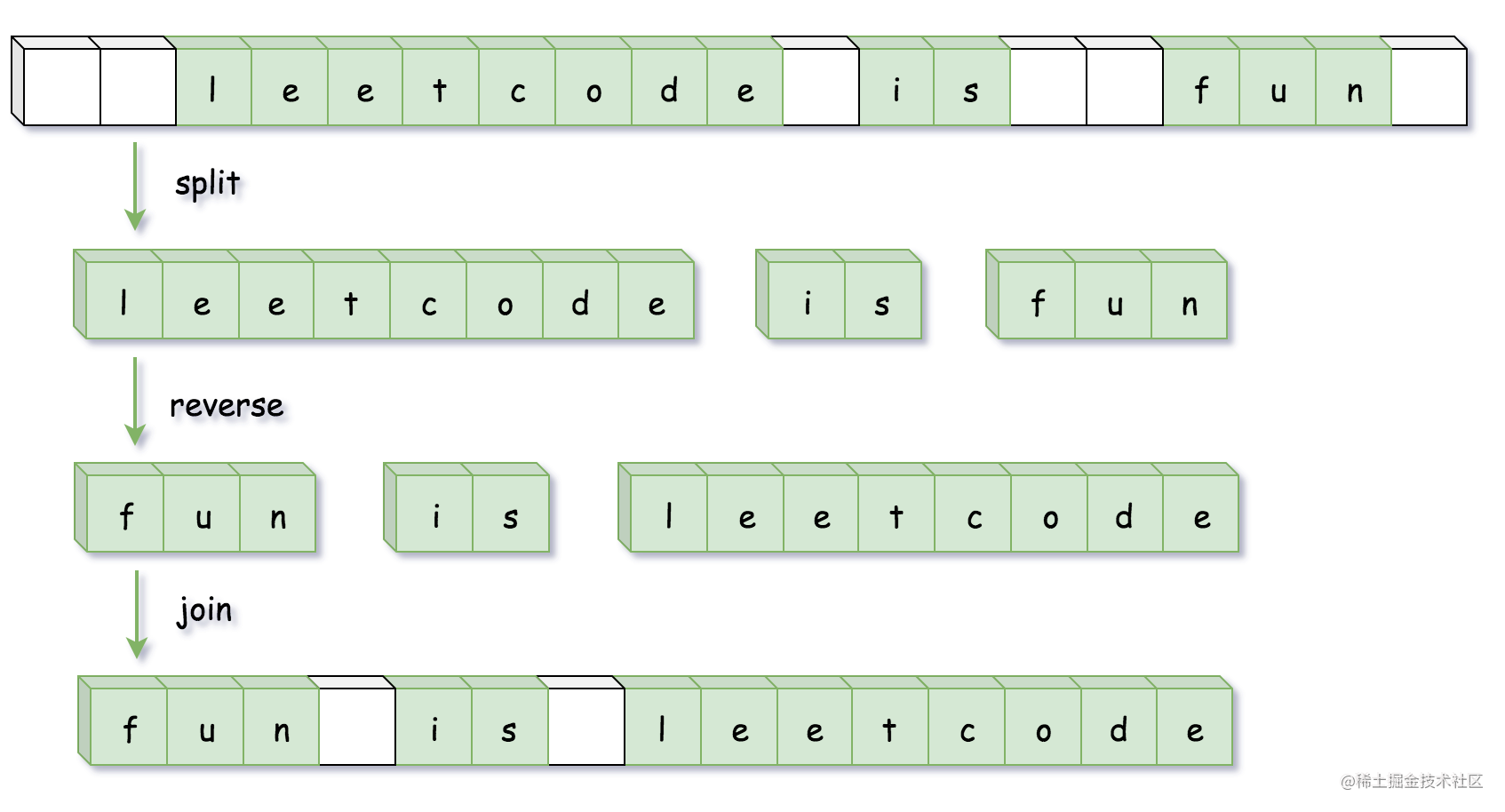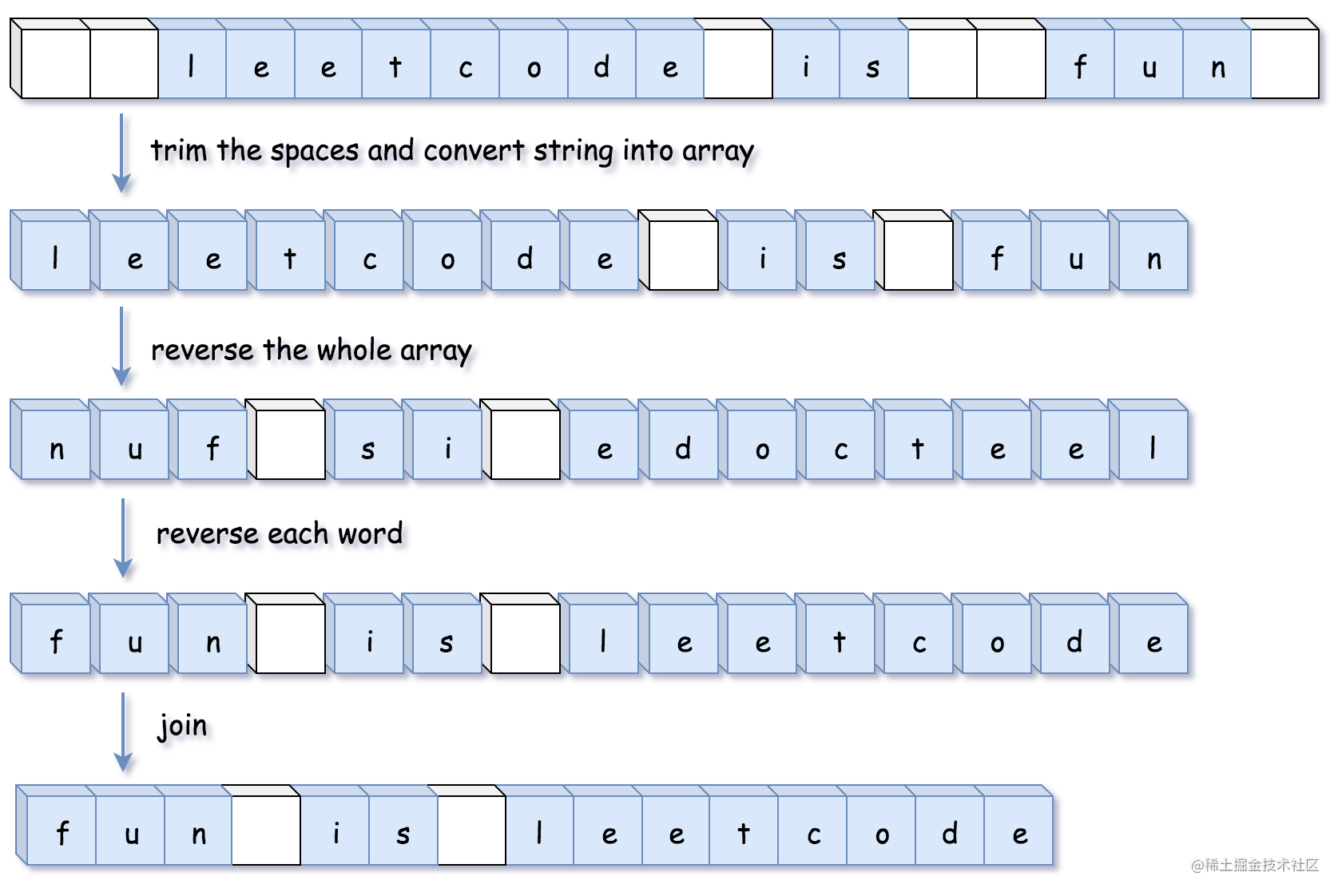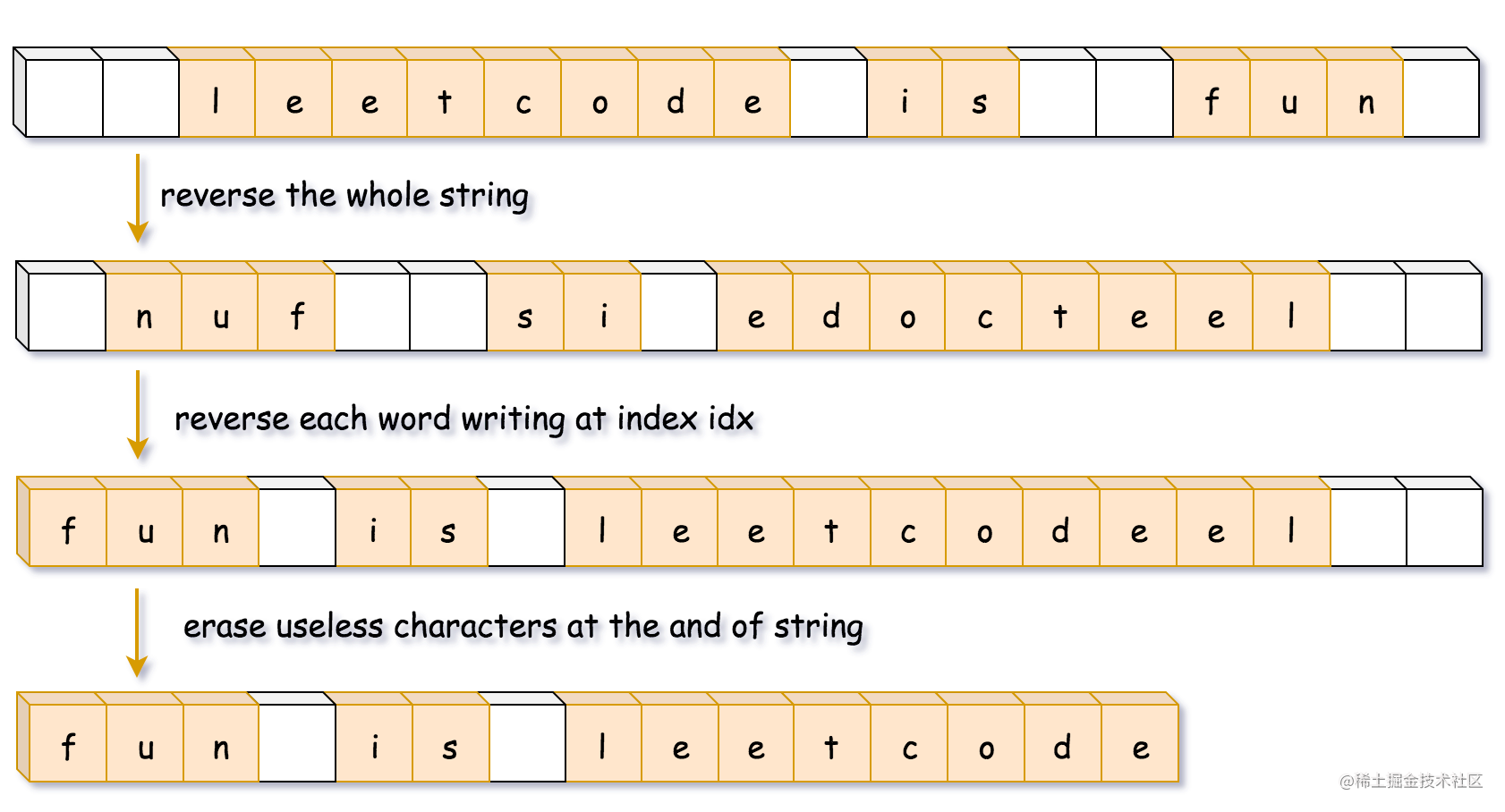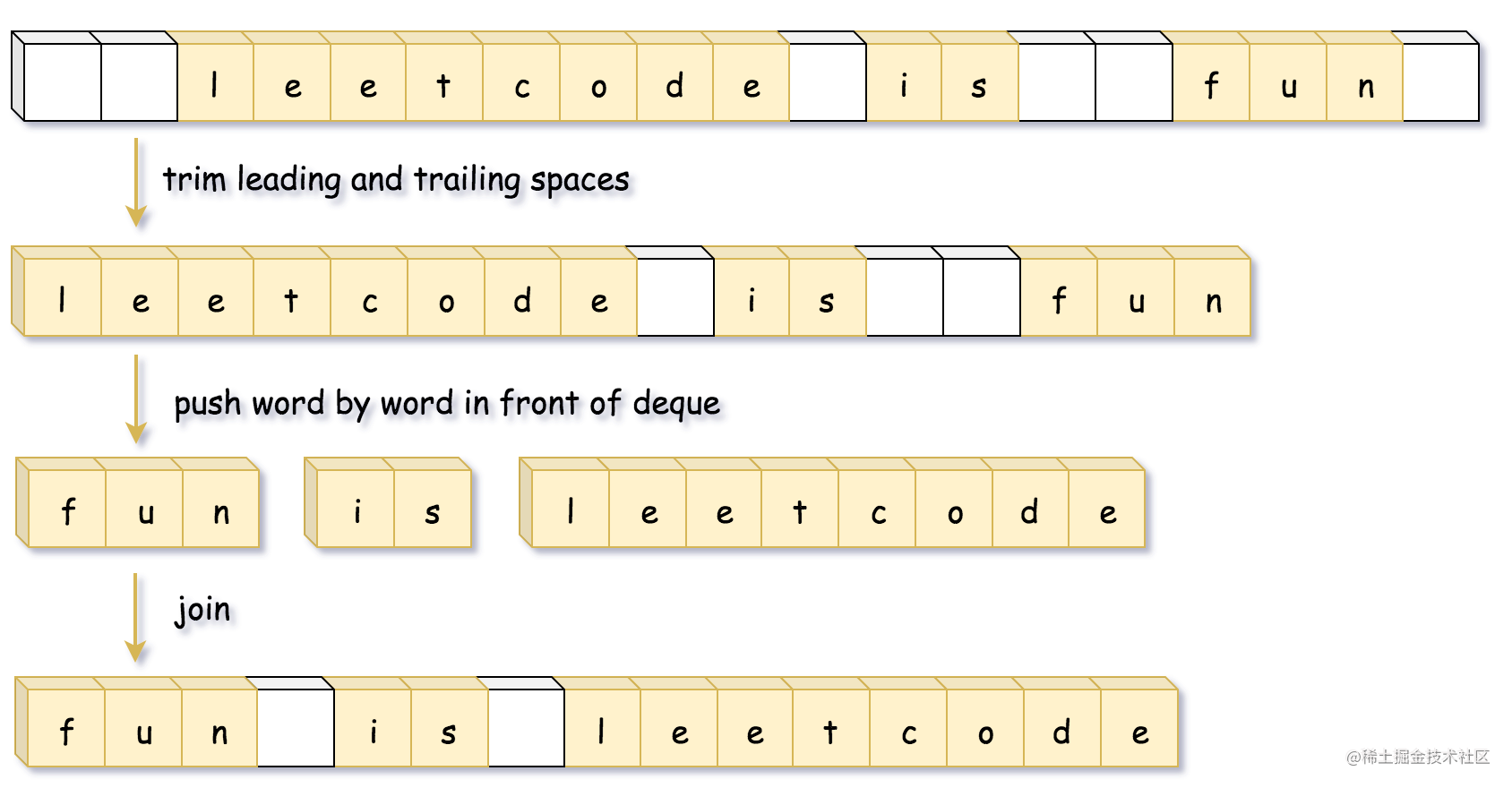# 分析

#### 方法一：使用语言特性

1. 使用 `split` 将字符串按空格分割成字符串数组；
2. 使用 `reverse` 将字符串数组进行反转；
3. 使用 `join` 方法将字符串数组拼成一个字符串。``````class Solution {
public String reverseWords(String s) {
// 除去开头和末尾的空白字符
s = s.trim();
// 正则匹配连续的空白字符作为分隔符分割
List<String> wordList = Arrays.asList(s.split("\\s+"));
Collections.reverse(wordList);
return String.join(" ", wordList);
}
}

• 时间复杂度：O(N)，其中 N 为输入字符串的长度。
• 空间复杂度：O(N)，用来存储字符串分割之后的结果。

#### 方法二：自行编写对应的函数``````class Solution {
public StringBuilder trimSpaces(String s) {
int left = 0, right = s.length() - 1;
// 去掉字符串开头的空白字符
while (left <= right && s.charAt(left) == ' ') ++left;

// 去掉字符串末尾的空白字符
while (left <= right && s.charAt(right) == ' ') --right;

// 将字符串间多余的空白字符去除
StringBuilder sb = new StringBuilder();
while (left <= right) {
char c = s.charAt(left);

if (c != ' ') sb.append(c);
else if (sb.charAt(sb.length() - 1) != ' ') sb.append(c);

++left;
}
return sb;
}

public void reverse(StringBuilder sb, int left, int right) {
while (left < right) {
char tmp = sb.charAt(left);
sb.setCharAt(left++, sb.charAt(right));
sb.setCharAt(right--, tmp);
}
}

public void reverseEachWord(StringBuilder sb) {
int n = sb.length();
int start = 0, end = 0;

while (start < n) {
// 循环至单词的末尾
while (end < n && sb.charAt(end) != ' ') ++end;
// 翻转单词
reverse(sb, start, end - 1);
// 更新start，去找下一个单词
start = end + 1;
++end;
}
}

public String reverseWords(String s) {
StringBuilder sb = trimSpaces(s);

// 翻转字符串
reverse(sb, 0, sb.length() - 1);

// 翻转每个单词
reverseEachWord(sb);

return sb.toString();
}
}

• 时间复杂度：O(N)，其中 N 为输入字符串的长度。
• 空间复杂度：`Java``Python` 的方法需要 O(N)O(N) 的空间来存储字符串，而 `C++` 方法只需要 `O(1)` 的额外空间来存放若干变量。

#### 方法三：双端队列``````class Solution {
public String reverseWords(String s) {
int left = 0, right = s.length() - 1;
// 去掉字符串开头的空白字符
while (left <= right && s.charAt(left) == ' ') ++left;

// 去掉字符串末尾的空白字符
while (left <= right && s.charAt(right) == ' ') --right;

Deque<String> d = new ArrayDeque();
StringBuilder word = new StringBuilder();

while (left <= right) {
char c = s.charAt(left);
if ((word.length() != 0) && (c == ' ')) {
// 将单词 push 到队列的头部
d.offerFirst(word.toString());
word.setLength(0);
} else if (c != ' ') {
word.append(c);
}
++left;
}
d.offerFirst(word.toString());

return String.join(" ", d);
}
}

• 时间复杂度：O(N)，其中 N 为输入字符串的长度。
• 空间复杂度：O(N)，双端队列存储单词需要 O(N) 的空间。